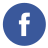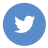### Java Numbers Class

java

In Java, the `java.lang.Number` class is the superclass of all classes that represent numeric values. It is an abstract class that provides common methods for converting numeric values to other types, such as `byte`, `short`, `int`, `long` , `float`, and `double`.

Here are some common methods of the `Number` class:

`byte byteValue()`: Returns the value of this Number as a byte.
`short shortValue()`: Returns the value of this Number as a short.
`int intValue()`: Returns the value of this Number as an int.
`long longValue()`: Returns the value of this Number as a long.
`float floatValue()`: Returns the value of this Number as a float.
`double doubleValue()`: Returns the value of this Number as a double.

Here is an example of how to use the `Number` class:

``````double d = 123.45;
Number n = d; // n is a Number object with the value 123.45

int i = n.intValue(); // i is 123
long l = n.longValue(); // l is 123
float f = n.floatValue(); // f is 123.45``````

Note that the `Number` class does not provide methods for arithmetic operations such as addition, subtraction, multiplication, and division. To perform arithmetic operations on numeric values, you can use the basic arithmetic operators discussed in a previous answer.